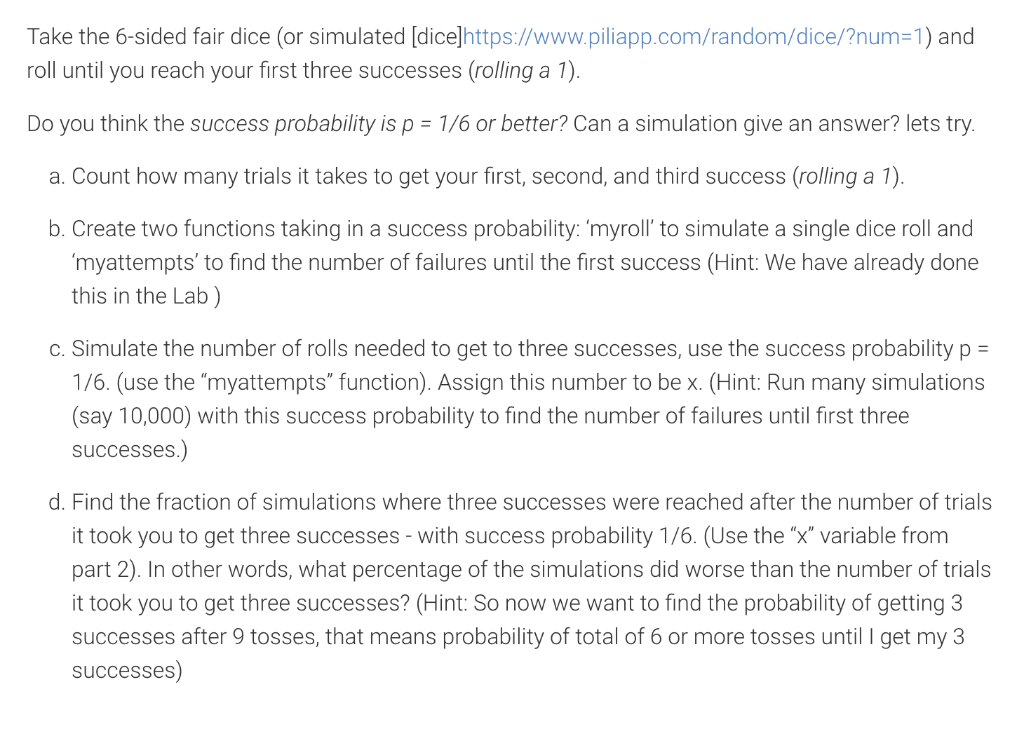Home / Expert Answers / Computer Science / thank-you-take-the-6-sided-fair-dice-or-simulated-dice-https-www-piliapp-com-random-dice-num-pa958

# (Solved): Thank you! Take the 6-sided fair dice (or simulated [dice]https://www.piliapp.com/random/dice/?num ...Thank you!

Take the 6-sided fair dice (or simulated [dice]https://www.piliapp.com/random/dice/?num=1) and roll until you reach your first three successes (rolling a 1). Do you think the success probability is \( p=1 / 6 \) or better? Can a simulation give an answer? lets try. a. Count how many trials it takes to get your first, second, and third success (rolling a 1). b. Create two functions taking in a success probability: 'myroll' to simulate a single dice roll and 'myattempts' to find the number of failures until the first success (Hint: We have already done this in the Lab ) c. Simulate the number of rolls needed to get to three successes, use the success probability \( p= \) 1/6. (use the "myattempts" function). Assign this number to be x. (Hint: Run many simulations (say 10,000) with this success probability to find the number of failures until first three successes.) d. Find the fraction of simulations where three successes were reached after the number of trials it took you to get three successes - with success probability \( 1 / 6 \). (Use the " \( x \) " variable from part 2). In other words, what percentage of the simulations did worse than the number of trials it took you to get three successes? (Hint: So now we want to find the probability of getting 3 successes after 9 tosses, that means probability of total of 6 or more tosses until I get my 3 successes)

We have an Answer from Expert